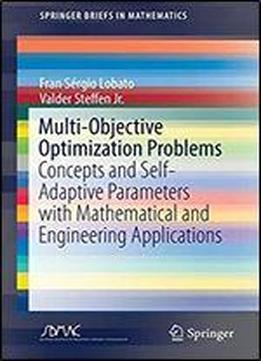# Multi-objective Optimization Problems: Concepts And Self-adaptive Parameters With Mathematical And Engineering Applications (springerbriefs In Mathematics) by Fran Sergio Lobato / 2017 / English / PDF

This book is aimed at undergraduate and graduate students in applied mathematics or computer science, as a tool for solving real-world design problems. The present work covers fundamentals in multi-objective optimization and applications in mathematical and engineering system design using a new optimization strategy, namely the Self-Adaptive Multi-objective Optimization Differential Evolution (SA-MODE) algorithm. This strategy is proposed in order to reduce the number of evaluations of the objective function through dynamic update of canonical Differential Evolution parameters (population size, crossover probability and perturbation rate). The methodology is applied to solve mathematical functions considering test cases from the literature and various engineering systems design, such as cantilevered beam design, biochemical reactor, crystallization process, machine tool spindle design, rotary dryer design, among others.

views: 346